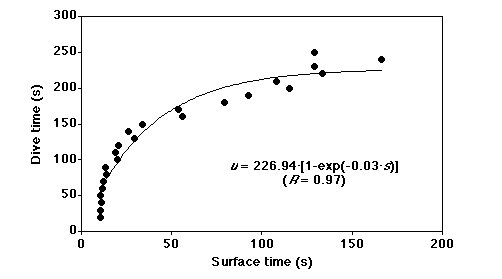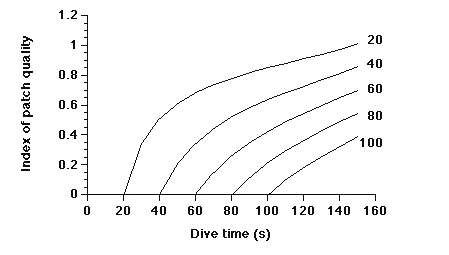### Yoshihisa Mori and Ian L. Boyd. 2004. The behavioral basis for nonlinear functional responses and its application to optimal foraging in Antarctic fur seals. Ecology 85:398–410.

Appendix A. Calculation of the index patch quality.

Dive time, u, consists of two variables: time spent in a foraging area (bottom time), f, and total time spent traveling from the surface to the foraging area, t.  The dive time is a function of the time spent on the water surface, s, because divers need time at the surface for recovery or replenishment from diving.  This function is likely to be an increasing but decelerating function (e.g., Kramer 1988, Houston and Carbone 1992, Mori 1998).  Thus, it follows that

 u = f + t = y(s), u = f + t = y(s), (A.1)

where y'(s) > 0 and y"(s) < 0.  Therefore, time necessary for foraging in fur seals is dive time plus surface time (dive cycle), u + s.  In the present study, y(s) (= u) was estimated as

 u = 227 [1 - exp(-0.03 s)]. (A.2)

This is the regression equation for dive time and the shortest succeeding surface time needed for the dive time obtained from the dive data (Fig. A1).

The amount of energy taken in during a dive cycle, g, depends on both time spent in a foraging area, f, and quality of the area such as prey density and prey type.  We assumed g to be a power function of time spent in a foraging area, f, or

 g = afx, (A.3)

after Mori et al. (2002), where parameter a represents a coefficient of conversion of the time spent at a foraging area into actual energy intake.  An exponent xrepresents the quality of a foraging area (Mori et al. 2002); larger exponent x gives larger amount of energy intake per unit time.  We call this exponent index of patch quality (IPQ).  Then net energy intake, G, during a dive cycle is

 G = g – (mff +  mtt + mss) = g – [mf(u – t) +  mtt + mss], (A.4)

where mf, mt, and ms are the energy consumption rates during times of f, t, and s, respectively.  Thus, net rate of energy intake during a dive cycle, E, is

 E = G/ (u + s). (A.5)

Metabolic rates during dive cycle (mf, mt, and ms) used in the calculation were based on empirical estimates.  Boyd et al. (1999) reported that metabolic rate of Antarctic fur seals did not differ between activities at sea and the average metabolic rate at sea was 5.71 J·s-1·kg-1.  A similar value (5.9 J·s-1·kg-1) was reported by Butler et al. (1995).  Thus, we set mf = mt = ms = 6.0 (J·s-1·kg-1) in Eq. A.4.

Since we set a constant metabolic rate among activities, in practice, the parameter a made no contribution to obtaining the IPQ.  Assuming that fur seals adjust their time allocation during dive cycle to maximize net rate of energy intake during a dive cycle, E, we could calculate the IPQ as follows for each dive from observed dive time and travel time.  From Eq. A.2 we obtained the inverse function which gave surface interval from the dive time.  This function was substituted into Eq. A.5.  The Eq. A.5, then, is a function of dive time, u, travel time, t and IPQ, x.  Since dive time and travel time were given by observation and it was assumed that the dive time was optimal for a given travel time and IPQ to maximize E, we could obtain IPQ as the exponent x which maximized Eq. A.5 (Fig. A2).  We could not calculate the exponent x for dives longer than 227 s, because of the assumption from Eq. A.2 that the maximum dive time of fur seals was 227 s, so these were excluded from the analysis.  However, almost all dives (359024 of 364385 dives, 98.6 %) were shorter than 227 s, so the effect of this procedure was negligible.  Mathematica ver. 4.0.1.0 (Wolfram Research, Inc. Champaign, Illinois, USA) was used for the computation.

Although we did not need the parameter a to calculate the IPQ, this parameter was necessary to estimate energy intake during a dive using Eq. A.3.  In Eq. A.3, we set a = 1600 (J·s-1·kg-1) based on the following assumptions.  Total energy intake during a breeding season (120 days) is ca. 3000 MJ and the proportion of time at sea was 66 % (80 days) of the total time (Arnould 1997).  Proportion of time spent submerged while at sea was 10.7 % (Boyd and Croxall 1992) and proportion of bottom time during a dive was 48 % (this study).  Assuming that the body weight of female Antarctic fur seals was 40 kg (e.g., Boyd et al. 1997), these figures give 211 (J·s-1·kg-1) as average energy intake rate during bottom time.  The present model assumes a power function for energy intake so that, when average bottom time is 30 s and average IPQ is ca. 0.4 (this study), the parameter a should satisfy a × 300.4 = 211 × 30, which gives a = 1624.  This parameter may be affected by prey species, but in the present study, our fur seals feed mainly on a single prey species (Antarctic krill).  Therefore, we assumed that the parameter a is constant regardless of foraging condition.FIG. A1. Dive time, u, and the shortest surface time, s, for the dive time. The regression curve is presented with its equation and its R value.FIG. A2. The relationship between dive time and the index of patch quality for various travel times in the study. Values shown against each line are travel times.

Literature cited

Arnould, J. P.Y. 1997.  Lactation and the cost of pup-rearing in Antarctic fur seal. Marine Mammal Science 13:516–526.

Boyd, I. L., and J. P. Croxall.1992. Diving behaviour of lactating Antarctic fur seals. Journal of Canadian Zoology 70:919–928.

Boyd, I. L., D. J. McCafferty, and T. R. Walker.1997. Variation in foraging effort by lactating Antarctic fur seals: response to simulated increased foraging costs. Behavioural Ecology and Sociobiology 40:135–144.

Boyd, I. L., R. M. Bevan, A. J. Woakes, and P. J. Butler.1999. Heart rate and behavior of fur seals: implications for measurement of field energetics.  American Journal of Physiology 276(Heart Circulation Physiology 45):H844–H857.

Butler, P. J., R. M. Bevan, A. J. Woakes, J. P. Croxall, and I. L. Boyd.1995. The use of data loggers to determine the energetics and physiology of aquatic birds and mammals. Brazilian Journal of Medical and Biological Research 28:1307–1317.

Houston, A. I., and C. Carbone.1992. The optimal allocation of time during the diving cycle. Behavioural Ecology 3:255–265.

Krammer, D. L.1988. The Behavioural ecology of air breathing by aquatic animals. Canadian Journal of Zoology 66:89–94.

Mori, Y.1998. Optimal choice of foraging depth in divers. Journal of Zoology, London 245:279–283.

Mori, Y., A. Takahashi, F. Mehlum, and Y. Watanuki. 2002. An application of optimal diving models to diving Behaviour of Brünnich's guillemots. Animal Behaviour 64:737–743.

[Back to E085-008]